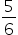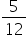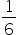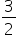Mathematics
Easy

Question

# If two dice are thrown, then the probability of getting the sum as a prime number is____.

##Hint:

## The correct answer is:### Here, we have to find the probability of getting a prime number on adding the outcomes when two dice are thrown.Total number of outcomes = 36Number of favourable outcome = 15Therefore, P( sum as prime) = 15/36= 5/12Hence, the correct option is (b).

0 ≤ P(E) ≤ 1 . Sum of probabilities of all events = 1#### With Turito Foundation.#### Get an Expert Advice From Turito.